# Resources tagged with: Addition & subtraction

Filter by: Content type:
Age range:
Challenge level:

### There are 227 results

Broad Topics > Calculations and Numerical Methods > Addition & subtraction### Magic Letters

##### Age 11 to 14Challenge Level

Charlie has made a Magic V. Can you use his example to make some more? And how about Magic Ls, Ns and Ws?### Have You Got It?

##### Age 11 to 14Challenge Level

Can you explain the strategy for winning this game with any target?### Summing Consecutive Numbers

##### Age 11 to 14Challenge Level

15 = 7 + 8 and 10 = 1 + 2 + 3 + 4. Can you say which numbers can be expressed as the sum of two or more consecutive integers?### Number Pyramids

##### Age 11 to 14Challenge Level

Try entering different sets of numbers in the number pyramids. How does the total at the top change?### Got it Article

##### Age 7 to 14

This article gives you a few ideas for understanding the Got It! game and how you might find a winning strategy.### Clocked

##### Age 11 to 14Challenge Level

Is it possible to rearrange the numbers 1,2......12 around a clock face in such a way that every two numbers in adjacent positions differ by any of 3, 4 or 5 hours?### Card Trick 2

##### Age 11 to 14Challenge Level

Can you explain how this card trick works?### Cubes Within Cubes

##### Age 7 to 14Challenge Level

We start with one yellow cube and build around it to make a 3x3x3 cube with red cubes. Then we build around that red cube with blue cubes and so on. How many cubes of each colour have we used?### Cunning Card Trick

##### Age 11 to 14Challenge Level

Delight your friends with this cunning trick! Can you explain how it works?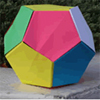### Dodecamagic

##### Age 7 to 11Challenge Level

Here you see the front and back views of a dodecahedron. Each vertex has been numbered so that the numbers around each pentagonal face add up to 65. Can you find all the missing numbers?### Aba

##### Age 11 to 14Challenge Level

In the following sum the letters A, B, C, D, E and F stand for six distinct digits. Find all the ways of replacing the letters with digits so that the arithmetic is correct.### Crossed Ends

##### Age 11 to 14Challenge Level

Crosses can be drawn on number grids of various sizes. What do you notice when you add opposite ends?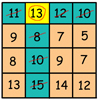### Making Maths: Be a Mathemagician

##### Age 7 to 11Challenge Level

Surprise your friends with this magic square trick.### Countdown Fractions

##### Age 11 to 16Challenge Level

Here is a chance to play a fractions version of the classic Countdown Game.### Consecutive Negative Numbers

##### Age 11 to 14Challenge Level

Do you notice anything about the solutions when you add and/or subtract consecutive negative numbers?### Multiply the Addition Square

##### Age 11 to 14Challenge Level

If you take a three by three square on a 1-10 addition square and multiply the diagonally opposite numbers together, what is the difference between these products. Why?### Calendar Capers

##### Age 11 to 14Challenge Level

Choose any three by three square of dates on a calendar page...### Charitable Pennies

##### Age 7 to 14Challenge Level

Investigate the different ways that fifteen schools could have given money in a charity fundraiser.### Eleven

##### Age 11 to 14Challenge Level

Replace each letter with a digit to make this addition correct.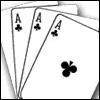### Amazing Card Trick

##### Age 11 to 14Challenge Level

How is it possible to predict the card?### Got it for Two

##### Age 7 to 14Challenge Level

Got It game for an adult and child. How can you play so that you know you will always win?### Magic Squares for Special Occasions

##### Age 11 to 16

This article explains how to make your own magic square to mark a special occasion with the special date of your choice on the top line.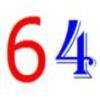### It's All about 64

##### Age 7 to 11Challenge Level

Write the numbers up to 64 in an interesting way so that the shape they make at the end is interesting, different, more exciting ... than just a square.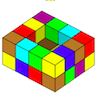### Painting Possibilities

##### Age 7 to 11Challenge Level

This task, written for the National Young Mathematicians' Award 2016, involves open-topped boxes made with interlocking cubes. Explore the number of units of paint that are needed to cover the boxes. . . .### Arrange the Digits

##### Age 11 to 14Challenge Level

Can you arrange the digits 1,2,3,4,5,6,7,8,9 into three 3-digit numbers such that their total is close to 1500?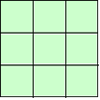### 1, 2, 3 Magic Square

##### Age 7 to 11Challenge Level

Arrange three 1s, three 2s and three 3s in this square so that every row, column and diagonal adds to the same total.### Largest Number

##### Age 11 to 14Challenge Level

What is the largest number you can make using the three digits 2, 3 and 4 in any way you like, using any operations you like? You can only use each digit once.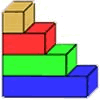### Rod Measures

##### Age 7 to 11Challenge Level

Using 3 rods of integer lengths, none longer than 10 units and not using any rod more than once, you can measure all the lengths in whole units from 1 to 10 units. How many ways can you do this?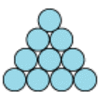### Difference

##### Age 7 to 11Challenge Level

Place the numbers 1 to 10 in the circles so that each number is the difference between the two numbers just below it.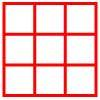### Ladybird Box

##### Age 5 to 11Challenge Level

Place six toy ladybirds into the box so that there are two ladybirds in every column and every row.### Double Digit

##### Age 11 to 14Challenge Level

Choose two digits and arrange them to make two double-digit numbers. Now add your double-digit numbers. Now add your single digit numbers. Divide your double-digit answer by your single-digit answer. . . .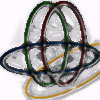### Bean Bags for Bernard's Bag

##### Age 7 to 11Challenge Level

How could you put eight beanbags in the hoops so that there are four in the blue hoop, five in the red and six in the yellow? Can you find all the ways of doing this?### Number Differences

##### Age 7 to 11Challenge Level

Place the numbers from 1 to 9 in the squares below so that the difference between joined squares is odd. How many different ways can you do this?### Up, Down, Flying Around

##### Age 11 to 14Challenge Level

Play this game to learn about adding and subtracting positive and negative numbers### Add to 200

##### Age 11 to 14Challenge Level

By selecting digits for an addition grid, what targets can you make?### Digit Addition

##### Age 5 to 11Challenge Level

Try out this number trick. What happens with different starting numbers? What do you notice?### Bundles of Cubes

##### Age 7 to 11Challenge Level

Watch this animation. What do you notice? What happens when you try more or fewer cubes in a bundle?### Forwards Add Backwards

##### Age 11 to 14Challenge Level

What happens when you add a three digit number to its reverse?### Subtraction Surprise

##### Age 7 to 14Challenge Level

Try out some calculations. Are you surprised by the results?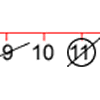### Strike it Out for Two

##### Age 5 to 11Challenge Level

Strike it Out game for an adult and child. Can you stop your partner from being able to go?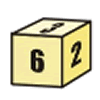### First Connect Three for Two

##### Age 7 to 11Challenge Level

First Connect Three game for an adult and child. Use the dice numbers and either addition or subtraction to get three numbers in a straight line.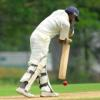### Twenty20

##### Age 7 to 16Challenge Level

Fancy a game of cricket? Here is a mathematical version you can play indoors without breaking any windows.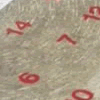### All Seated

##### Age 7 to 11Challenge Level

Look carefully at the numbers. What do you notice? Can you make another square using the numbers 1 to 16, that displays the same properties?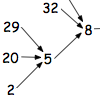### Follow the Numbers

##### Age 7 to 11Challenge Level

What happens when you add the digits of a number then multiply the result by 2 and you keep doing this? You could try for different numbers and different rules.### Making Maths: Double-sided Magic Square

##### Age 7 to 14Challenge Level

Make your own double-sided magic square. But can you complete both sides once you've made the pieces?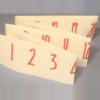### Folded Number Line

##### Age 7 to 11Challenge Level

When I fold a 0-20 number line, I end up with 'stacks' of numbers on top of each other. These challenges involve varying the length of the number line and investigating the 'stack totals'.### Diagonal Sums

##### Age 7 to 14Challenge Level

In this 100 square, look at the green square which contains the numbers 2, 3, 12 and 13. What is the sum of the numbers that are diagonally opposite each other? What do you notice?### Adding All Nine

##### Age 11 to 14Challenge Level

Make a set of numbers that use all the digits from 1 to 9, once and once only. Add them up. The result is divisible by 9. Add each of the digits in the new number. What is their sum? Now try some. . . .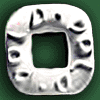### Polo Square

##### Age 7 to 11Challenge Level

Arrange eight of the numbers between 1 and 9 in the Polo Square below so that each side adds to the same total.### A Square of Numbers

##### Age 7 to 11Challenge Level

Can you put the numbers 1 to 8 into the circles so that the four calculations are correct?